# Common Core: 6th Grade Math : Write and Evaluate Numerical Expressions With Exponents: CCSS.Math.Content.6.EE.A.1

## Example Questions

← Previous 1 3 4 5 6 7 8 9 10 11

### Example Question #82 : Equations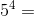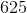Explanation:

Multiply: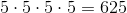Answer:### Example Question #2 : Write And Evaluate Numerical Expressions With Exponents: Ccss.Math.Content.6.Ee.A.1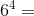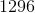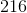Explanation:

Multiply: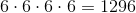Answer:### Example Question #3 : Write And Evaluate Numerical Expressions With Exponents: Ccss.Math.Content.6.Ee.A.1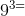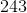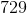Explanation:

Multiply: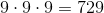Answer:### Example Question #4 : Write And Evaluate Numerical Expressions With Exponents: Ccss.Math.Content.6.Ee.A.1

Simplify: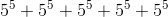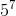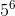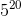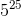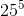Explanation:

When adding exponents, we first need to factor common terms.

Let's start by factoring out the following: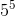Factor.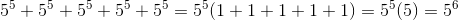### Example Question #5 : Write And Evaluate Numerical Expressions With Exponents: Ccss.Math.Content.6.Ee.A.1

Expand: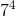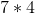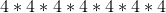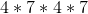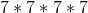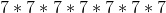Explanation:

When a number is raised by an exponent, the base value is multiplied by itself the number of times that the exponential value indicates.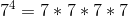### Example Question #6 : Write And Evaluate Numerical Expressions With Exponents: Ccss.Math.Content.6.Ee.A.1

Expand: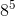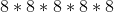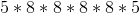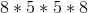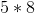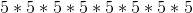Explanation:

When a number is raised by an exponent, the base value is multiplied by itself the number of times that the exponential value indicates.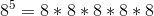### Example Question #1 : Write And Evaluate Numerical Expressions With Exponents: Ccss.Math.Content.6.Ee.A.1

Evaluate: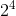Explanation:

When a number is raised by an exponent, the base value is multiplied by itself the number of times that the exponential value indicates.is expanded to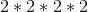.

The product is.

### Example Question #21 : Simple Exponents

Evaluate: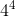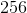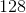Explanation:

When a number is raised by an exponent, the base value is multiplied by itself the number of times that the exponential value indicates.is expanded to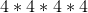.

The product is.

### Example Question #9 : Write And Evaluate Numerical Expressions With Exponents: Ccss.Math.Content.6.Ee.A.1

Expand: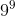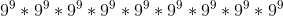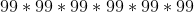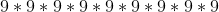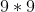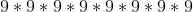Explanation:To expand the exponent, we just multiply the base out by the exponent present.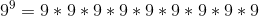### Example Question #1 : Expressions & Equations

Expand: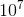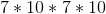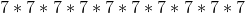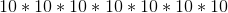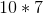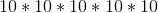Explanation:

To expand the exponent, we just multiply the base out by the exponent present.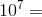← Previous 1 3 4 5 6 7 8 9 10 11

### All Common Core: 6th Grade Math Resources